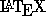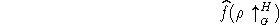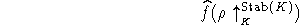Next: Working with Large Files Up: An Introduction to Using Previous: Diagrams

# Making Life a Little Easierprovides the typesetting capability to create virtually any mathematical expression you could ever want in a paper. Unfortunately, you may find yourself typing long strings of symbol commands over and over again. For example, representation theory people may find themselves typing something like

$$\widehat{f}(\rho\uparrow_{_G}^H)$$

to getIt would be nice if you could type a shorter string. You can do this by defining your own macro'' with a line like this

\newcommand{\fhatrhoinG}{\widehat{f}(\rho\uparrow_{_G}^H)}

Now every time you type (in math mode) the string

\fhatrhoinG

you get the above notation. Like the \newtheorem command, this line can appear anywhere in your file (before the first use of the macro it defines), but it is conventional to put it between the \documentstyle and \begin{document} lines.

You can also define commands which take parameters. For example, suppose that H and G might vary in the above example. Then the following macro can be defined:

\newcommand{\fhatrhoin}{\widehat{f}
(\rho\uparrow_{_{#1}}^{#2})}

The  means that there are two arguments. The two arguments are inserted in the macro's text where {#1} and {#2} appear. Thus, typing the command

$$\fhatrhoin{K}{\operatorname{Stab}(K)}$$

for example, will yieldWhile you're at it, you could also define

\newcommand{\Stab}{\operatorname{Stab}}
if you have stabilizers in your document.

Processed by LaTeX2html